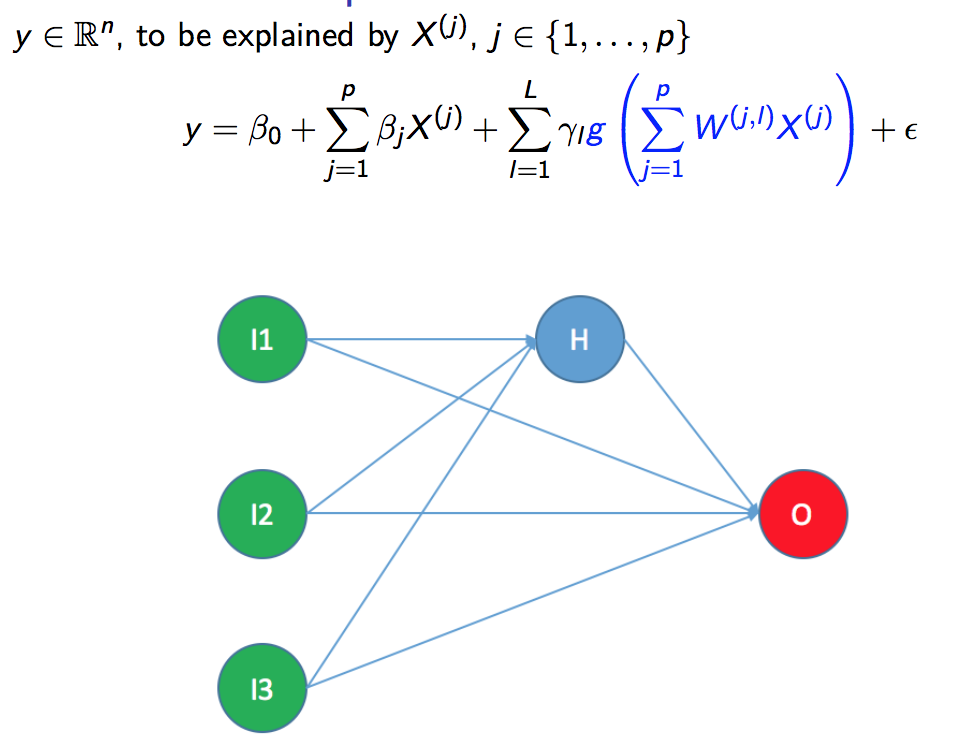A new version of nnetsauce, v0.10.0, is available on Pypi (for Python) and GitHub (for R). To those who’ve never heard about nnetsauce: it’s a package for supervised learning (as of February 2022, you can solve regression, classification, and time series forecasting problems with nnetsauce) based on various combinations of components $$g(XW+b)$$, with:

• $$X$$, a matrix of explanatory variables or multivariate (univariate works too, but hasn’t been tested enough yet) time series
• $$W$$, a matrix which contains quasirandom numbers, that help in achieving a kind of automated feature engineering ($$XW$$)
• $$b$$, a bias term
• $$g$$, an activation function, used for model nonlinearity (otherwise, without it, the model would be linear)

For example, here is how nnetsauce can be used to create a nonlinear model from a linear model:In nnetsauce v0.10.0, the most important change is a – potentially breakingchange in the API: classes’ attributes (mostly, computed in method fit) which do not belong to the interface have been renamed with a suffix “_”. As in scikit-learn.

Multiple Python examples can be found on GitHub, along with notebooks. Here is an example of use of the package in R (on Ubuntu):

library(devtools)
devtools::install_github("Techtonique/nnetsauce/R-package")
library(nnetsauce)

set.seed(123)
(n <- nrow(iris))
(index_train <- sample.int(n, size = floor(0.8*n), replace = FALSE))

X_train <- as.matrix(iris[index_train, 1:4])
y_train <- as.integer(iris[index_train, 5]) - 1L
X_test <- as.matrix(iris[-index_train, 1:4])
y_test <- as.integer(iris[-index_train, 5]) - 1L

print(obj$get_params()) obj$fit(X_train, y_train)
print(obj\$score(X_test, y_test)) # accuracy


R session info:

> sessionInfo()
R version 4.1.2 (2021-11-01)
Platform: x86_64-pc-linux-gnu (64-bit)
Running under: Ubuntu 20.04.3 LTS

Matrix products: default
BLAS:   /usr/lib/x86_64-linux-gnu/atlas/libblas.so.3.10.3
LAPACK: /usr/lib/x86_64-linux-gnu/atlas/liblapack.so.3.10.3

locale:
 LC_CTYPE=C.UTF-8       LC_NUMERIC=C           LC_TIME=C.UTF-8
 LC_COLLATE=C.UTF-8     LC_MONETARY=C.UTF-8    LC_MESSAGES=C.UTF-8
 LC_TELEPHONE=C         LC_MEASUREMENT=C.UTF-8 LC_IDENTIFICATION=C

attached base packages:
 stats     graphics  grDevices utils     datasets  methods   base

other attached packages:
 nnetsauce_0.10.0

loaded via a namespace (and not attached):
 Rcpp_1.0.8       magrittr_2.0.2   rappdirs_0.3.3   munsell_0.5.0    colorspace_2.0-2
 here_1.0.1       lattice_0.20-45  R6_2.5.1         rlang_1.0.1      fansi_1.0.2
 tools_4.1.2      grid_4.1.2       gtable_0.3.0     png_0.1-7        utf8_1.2.2
 cli_3.1.1        ellipsis_0.3.2   rprojroot_2.0.2  tibble_3.1.6     lifecycle_1.0.1
 crayon_1.4.2     Matrix_1.3-4     ggplot2_3.3.5    vctrs_0.3.8      glue_1.6.1
 compiler_4.1.2   pillar_1.7.0     scales_1.1.1     reticulate_1.24  jsonlite_1.7.3
 pkgconfig_2.0.3